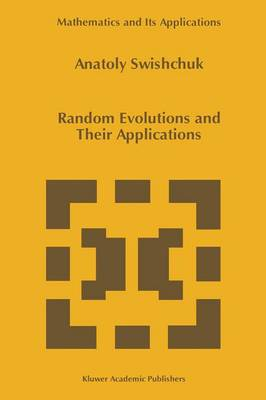•# Random Evolutions and Their Applications - Mathematics and Its Applications 408 (Hardback)

(author)
£67.99
Hardback 183 Pages / Published: 30/04/1997
• We can order this

Usually dispatched within 3 weeks

The main purpose of this handbook is to summarize and to put in order the ideas, methods, results and literature on the theory of random evolutions and their applications to the evolutionary stochastic systems in random media, and also to present some new trends in the theory of random evolutions and their applications. In physical language, a random evolution ( RE ) is a model for a dynamical sys tem whose state of evolution is subject to random variations. Such systems arise in all branches of science. For example, random Hamiltonian and Schrodinger equations with random potential in quantum mechanics, Maxwell's equation with a random refractive index in electrodynamics, transport equations associated with the trajec tory of a particle whose speed and direction change at random, etc. There are the examples of a single abstract situation in which an evolving system changes its "mode of evolution" or "law of motion" because of random changes of the "environment" or in a "medium". So, in mathematical language, a RE is a solution of stochastic operator integral equations in a Banach space. The operator coefficients of such equations depend on random parameters. Of course, in such generality , our equation includes any homogeneous linear evolving system. Particular examples of such equations were studied in physical applications many years ago. A general mathematical theory of such equations has been developed since 1969, the Theory of Random Evolutions.

Publisher: Springer
ISBN: 9780792345336
Number of pages: 183
Weight: 1080 g
Dimensions: 235 x 155 x 14 mm
Edition: 1997 ed.